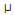# Electronics - Resistance and Power

### Exercise :: Resistance and Power - General Questions

26.

How many basic types of resistors exist?

 A. 1 B. 2 C. 3 D. 4

Explanation:

No answer description available for this question. Let us discuss.

27.

With a complex circuit, a supply source senses:

 A. open circuit components B. when voltages need to be increased C. only a single resistive connection D. when complex currents are needed

Explanation:

No answer description available for this question. Let us discuss.

28.

How many ohms of resistance allows a current of 720A to flow when 3.6 kV is applied?

 A. 200 nB. 5 kC. 200 kD. 5 MExplanation:

No answer description available for this question. Let us discuss.

29.

Which is the most important step utilized when measuring resistors?

 A. use the highest possible scale B. keep test leads short C. zero the meter before using D. remove power from the circuit

Explanation:

No answer description available for this question. Let us discuss.

30.

Components designed to oppose the flow of current are called:

 A. insulators B. conductors C. resistors D. heat exchangers